# Reciprocal

Calculate reciprocal of z=0.8-1.8i:

Re =  0.21
Im =  0.46

### Step-by-step explanation: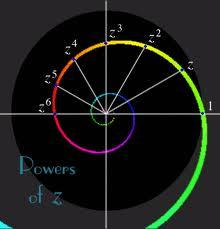Did you find an error or inaccuracy? Feel free to write us. Thank you!Tips to related online calculators

## Related math problems and questions:

• Reciprocal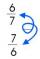Calculate the reciprocal numbers for the given real numbers.
• De Moivre's formula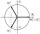There are two distinct complex numbers z, such that z3 is equal to 1 and z is not equal to 1. Calculate the sum of these two numbers.
• Reciprocal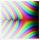What is the reciprocal of the sum of the reciprocals of 7 and 6?
• ABS CNCalculate the absolute value of complex number -15-29i.
• Let z1=x1+y1i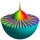Let z1=x1+y1i and z2=x2+y2i Find: a = Im (z1z2) b = Re (z1/z2)
• Reciprocal value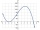How do I calculate a number x that is 9 greater than its reciprocal (1/x)?
• Is complexAre these numbers 2i, 4i, 2i + 1, 8i, 2i + 3, 4 + 7i, 8i, 8i + 4, 5i, 6i, 3i complex?
• The modulus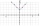Find the modulus of the complex number 2 + 5i
• Distance two imaginary numbs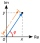Find the distance between two complex number: z1=(-8+i) and z2=(-1+i).
• Goniometric form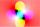Determine the goniometric form of a complex number z = √ 110 +4 i.
• Mappings of complex numbersFind the images of the following points under mappings: z=3-2j w=2zj+j-1
• Log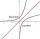Calculate value of expression log |3 +7i +5i2| .
• Linear imaginary equationGiven that 2(z+i)=i(z+i) "this is z star" Find the value of the complex number z.
• Complex number coordinatesWhich coordinates show the location of -2+3i
• Linear combination of complex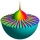If z1=5+3i and z2=4-2i, write the following in the form a+bi a) 4z1+6z2 b) z1*z2
• Eq with reciprocalSolve given equation with reciprocal member: a-6/a+10=4/8
• Im>0?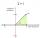Is -10i a positive number?